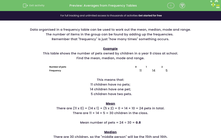# Averages from Frequency Tables

In this worksheet, students read data from frequency tables in order to work out the mean, median, mode and range.Key stage:  KS 3

Curriculum topic:   Statistics

Curriculum subtopic:   Understand Variables, Representation, Measures and Spread

Difficulty level:#### Worksheet Overview

Data organised in a frequency table can be used to work out the mean, median, mode and range.

The number of items in the group can be found by adding up the frequencies.

Remember that "frequency" is just "how many times" something occurs.

Example

This table shows the number of pets owned by children in a year 9 class at school.

Find the mean, median, mode and range.

Number of pets 0 1 2
Frequency 11 14 5

This means that:

11 children have no pets;

14 children have one pet;

5 children have two pets.

Mean

There are (11 x 0) + (14 x 1) + (5 x 2) = 0 + 14 + 10 = 24 pets in total.

There are 11 + 14 + 5 = 30 children in the class.

Mean number of pets = 24 ÷ 30 = 0.8

Median

There are 30 children, so the "middle person" will be the 15th and 16th.

The 15th and 16th person falls into the middle category and has 1 pet.

The median number of pets is 1

Mode

This is the one with the highest frequency.

Most children (14) have 1 pet.

The modal number of pets is 1

Range

The largest number of pets is 2 and the smallest is 0.

Range = 2 - 0 = 2

### What is EdPlace?

We're your National Curriculum aligned online education content provider helping each child succeed in English, maths and science from year 1 to GCSE. With an EdPlace account you’ll be able to track and measure progress, helping each child achieve their best. We build confidence and attainment by personalising each child’s learning at a level that suits them.

Get started